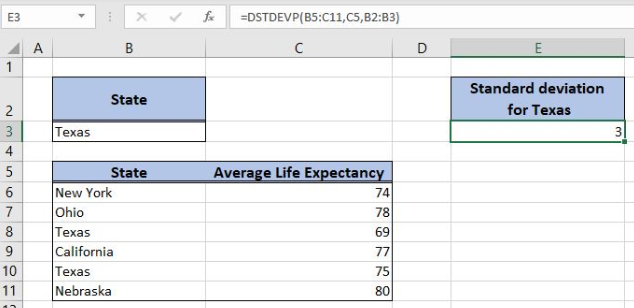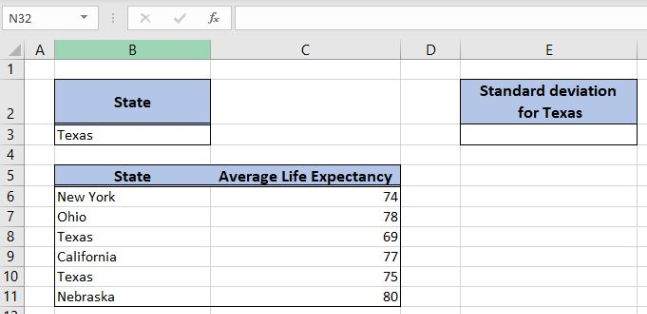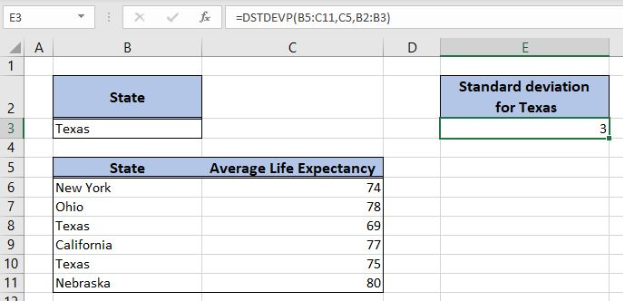Get instant live expert help with Excel or Google Sheets“My Excelchat expert helped me in less than 20 minutes, saving me what would have been 5 hours of work!”

#### Post your problem and you’ll get expert help in seconds.

Your message must be at least 40 characters
Our professional experts are available now. Your privacy is guaranteed.

# Excel ROUNDUP function

Excel allows us to round a decimal number up to a certain number of decimal places, by using the ROUNDUP function. This step by step tutorial will assist all levels of Excel users in rounding a decimal number up.Figure 1. The result of the ROUNDUP function

## Syntax of the ROUNDUP Formula

The generic formula for the ROUNDUP function is:

`=ROUNDUP(number, num_digits)`

The parameters of the ROUNDUP function are:

• number – a number which we want to round
• num_digits – a number of decimal places to round.

## Setting up Our Data for the ROUNDUP Function

In column B (“Number”), we have the list of numbers and in column C (“Number of decimal places”) we have a number of decimal places for rounding. In column C (“Rounded number”), we want to get a number rounded up.Figure 2. Data that we will use in the ROUNDUP example

## Round a Number Up

In our example, we want to round numbers up from column B to decimals in column C.

The formula in the cell D3 is:

`=ROUNDUP(B3, C3)`

The parameter number is the cell B3, while the num_digits is C3.

To apply the ROUNDUP function, we need to follow these steps:

• Select cell D3 and click on it
• Insert the formula: `=ROUNDUP(B3, C3)`
• Press enter
• Drag the formula down to the other cells in the column by clicking and dragging the little “+” icon at the bottom-right of the cell.Figure 3. Using the ROUNDUP function

When rounding the number, the function will always round a number up, regardless of the next decimal. We can see that in the cell D3, 2.34539 is rounded to 2.4. In the simple round, this number would be rounded to 2.3, because the next decimal is 4.

Most of the time, the problem you will need to solve will be more complex than a simple application of a formula or function. If you want to save hours of research and frustration, try our live Excelchat service! Our Excel Experts are available 24/7 to answer any Excel question you may have. We guarantee a connection within 30 seconds and a customized solution within 20 minutes.

### Did this post not answer your question? Get a solution from connecting with the expert.Another blog reader asked this question today on Excelchat:
Solution examplesI want to express any number with a fraction to roundup to the nearest 1/3, 2/3 or 1. Example: 121.001- 121.333= 121 1/3 121.334- 121.666= 121 2/3 121.667- 121.999= 122 OR IF CELL A1= 131.001- 131.333 THEN RETURN 131 1/3 IF CELL A1= 131.334- 131.666 THEN RETURN 131 2/3 IF CELL A1= 131.667- 131.999 THEN RETURN 132
Solved by E. Q. in 60 mins## Subscribe to Excelchat.coAnother blog reader asked this question today on Excelchat: